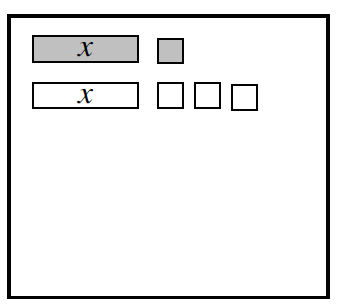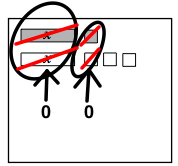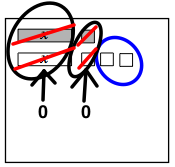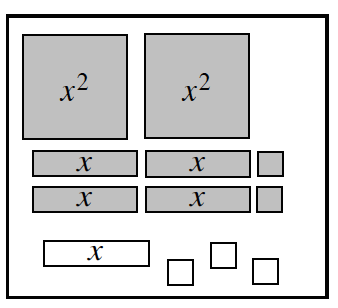### Home > CC2 > Chapter Ch6 > Lesson 6.1.1 > Problem6-6

6-6.

Write  the expression shown on each of the Expression Mats below. Then simplify them by making zeros and combining like terms.1.Notice that a grey tile and a white tile of the same value cancel each other out and make a zero. Circle around the positive x and the negative x tiles, with the tiles crossed out, labeled 0. A second circle around the positive unit and the negative unit tiles, with the tiles crossed out, labeled 0.

Now write an expression for the values that remain. A circle is added around the remaining 2 unit tiles.$−2$

1.See part (a).

$2x^2+3x-1$

Use the eTool below to solve part (a).
Click the link at right for the full version of the eTool: 6-6 HW eTool (CPM)